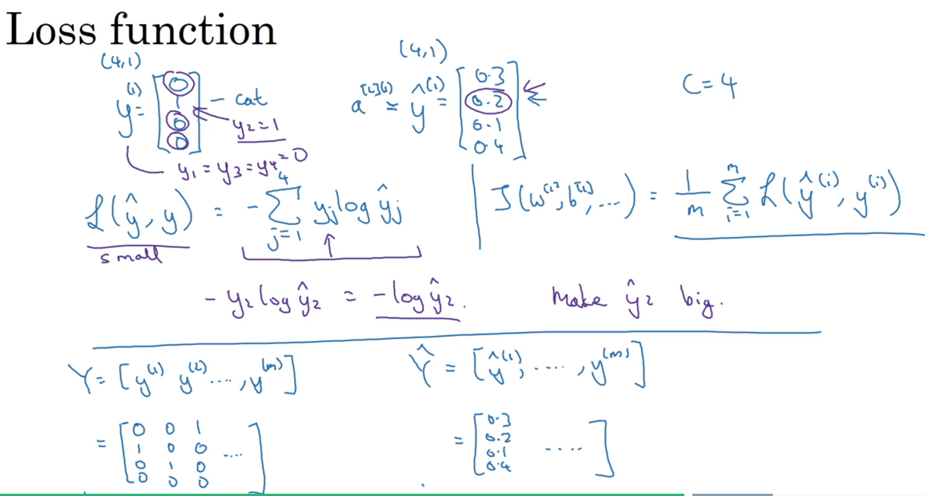## Evaluation

cross validation set也叫做dev set。在数据量不多时，可以按照60/20/20的比例来分配train/dev/test集合数据。但在数据很多时，比如一百万，百分之一就是一万，可以按照98/1/1来分配，并不需要那么多的dev/test。

1. train数据需要比较精确，准确度高。
2. dev/test数据可以相对不精确（这样才可以测试出学习的效果）
3. dev/test数据要保证同概率分布（我理解就是同源的意思，这样子用dev调教的结果才可以更好地验证test，如果两个不同分布，dev的调教结果就没有太大意义。）

• 先少量数据看是否有high bias，如果有，可以提高神经网络的层级，引入更多复杂的特征，或者调整神经网络的架构。（might help）
• 解决了bias，看有没有variance，如果有，可以引入更多的数据，regularization，或者调整神经网络架构（might help）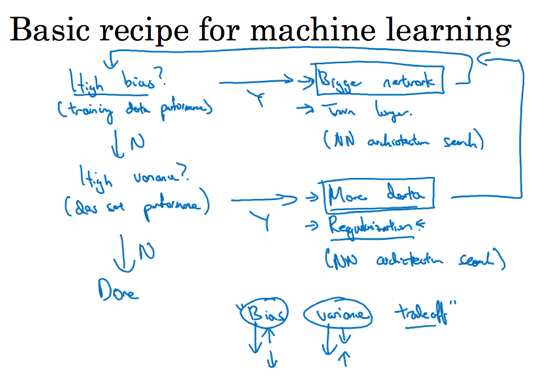## Regularization

regularization计算模型中引入两个术语：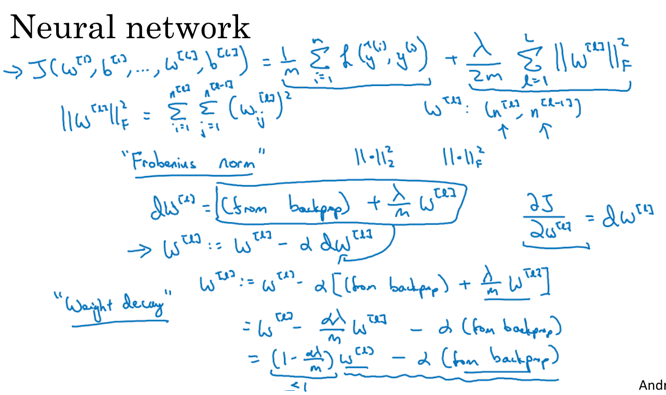• Frobenius norm：费罗贝尼乌斯范式，其实就是一个矩阵所有元素的L2距离求和。
• weight decay: regularization的计算其实就是一种weight decay，原来的weight加入regularization就等于先自减一个比例$1-{\alpha\lambda \over m}$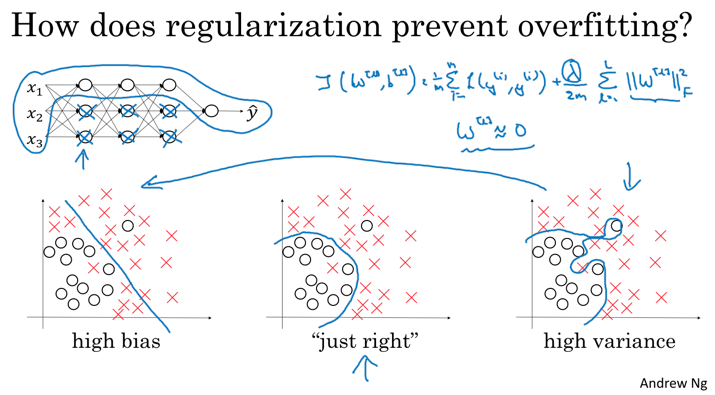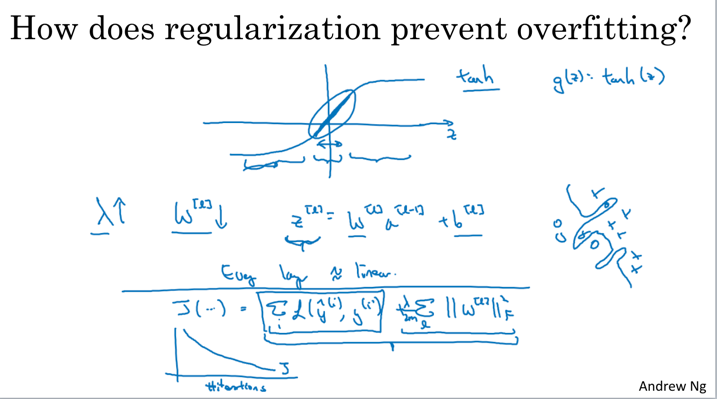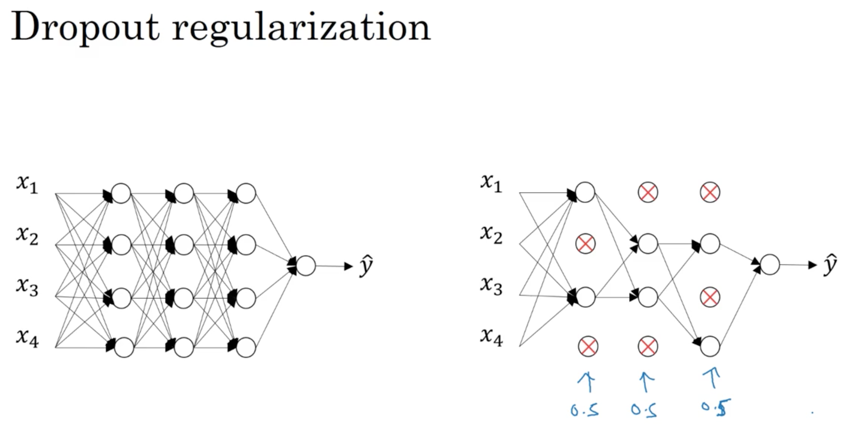dropout regularization计算的想法是：不要依赖任何一个特征，降低权重的方差。也就是说，让权重分布的更加均匀，而不依赖于相对权重较大的特征。所以计算时，随机去掉一些节点的计算结果，让所有节点对最后的权值起到平均的影响。

## Optimization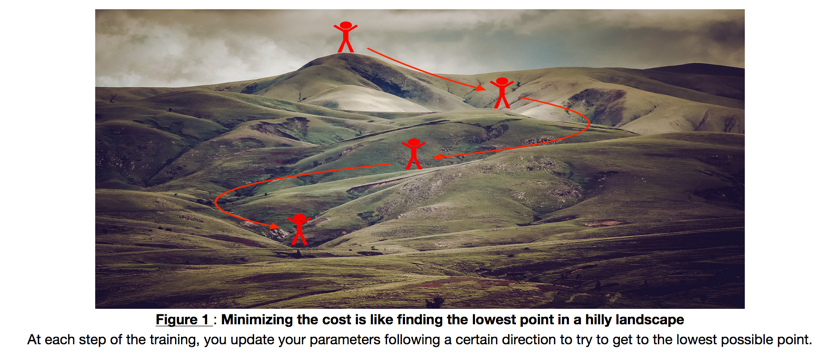### Initialization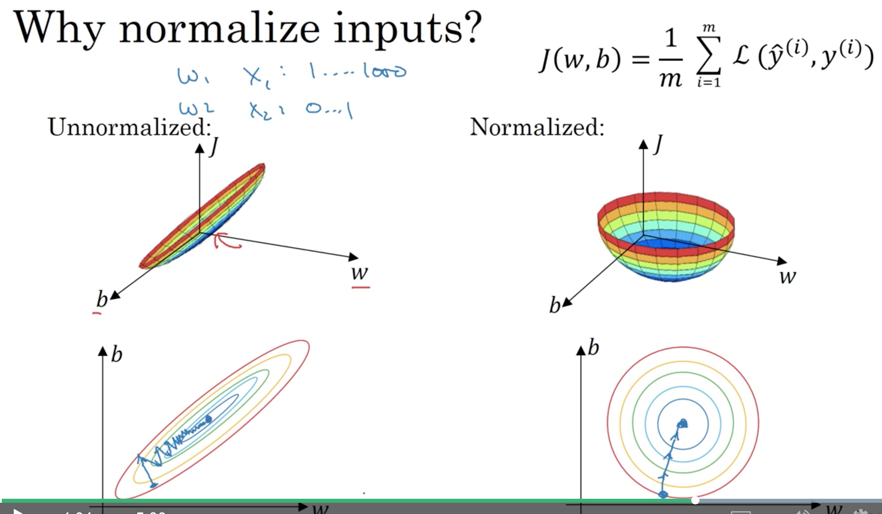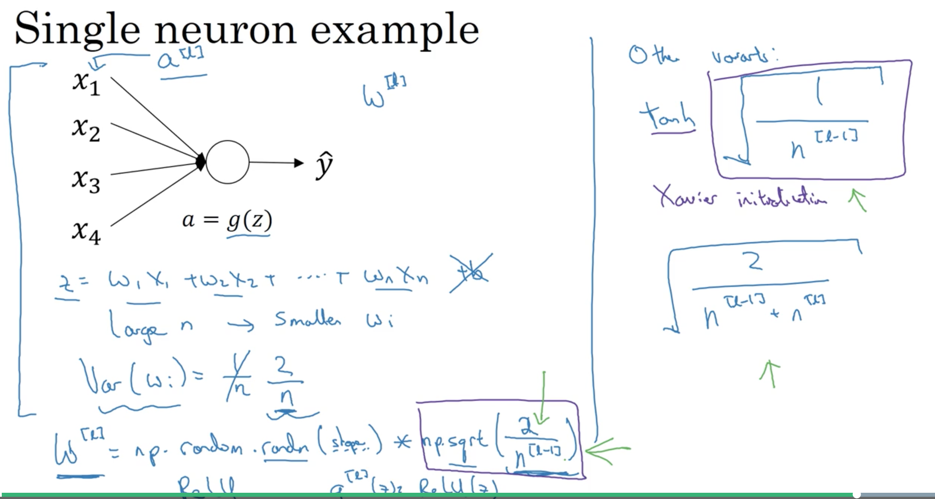### Mini-batch Gradient descent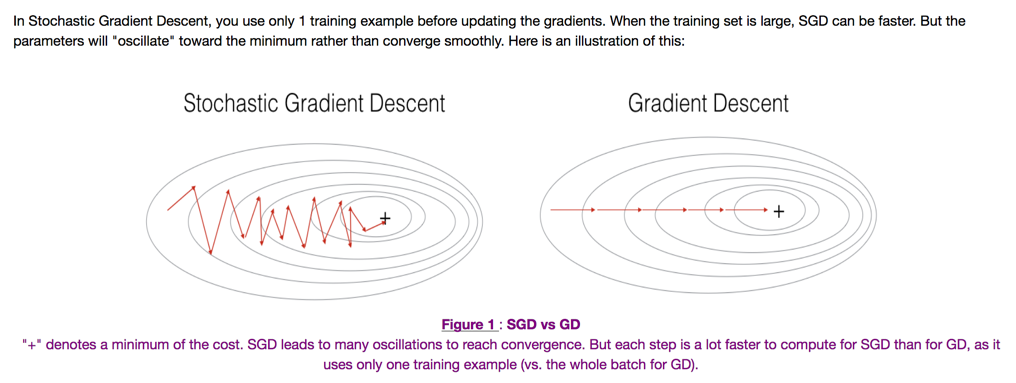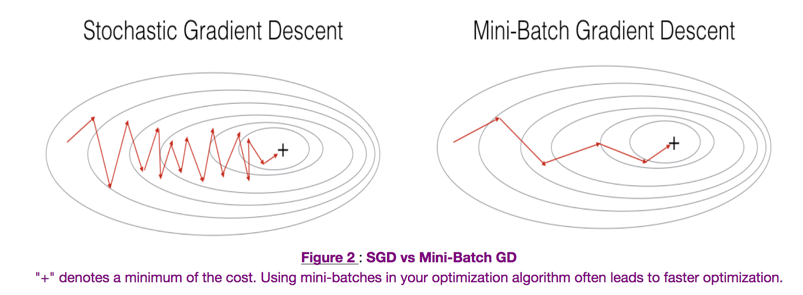### Gradient descent with Momentum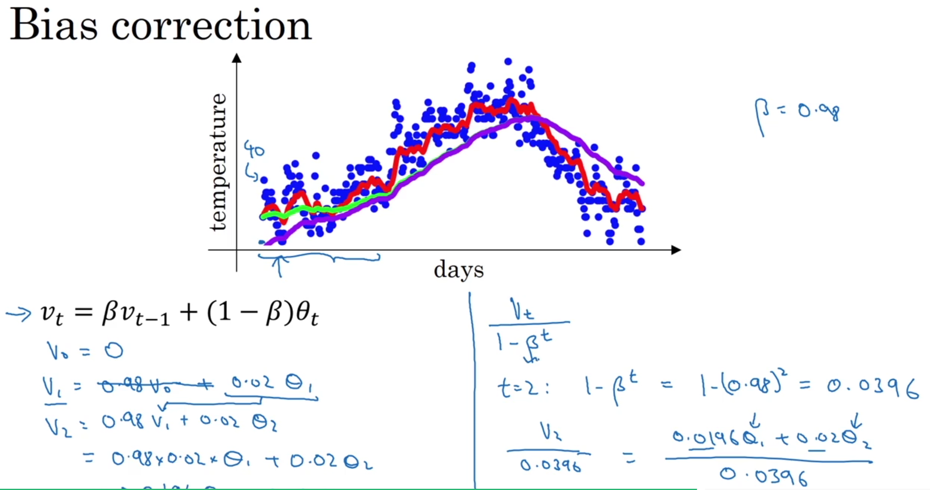$\sum_{i=0}^{t}{\beta^{t-i}{(1-\beta)}\theta_i}$

$v_t^{corrected} = {v_t \over {1-\beta^t}}$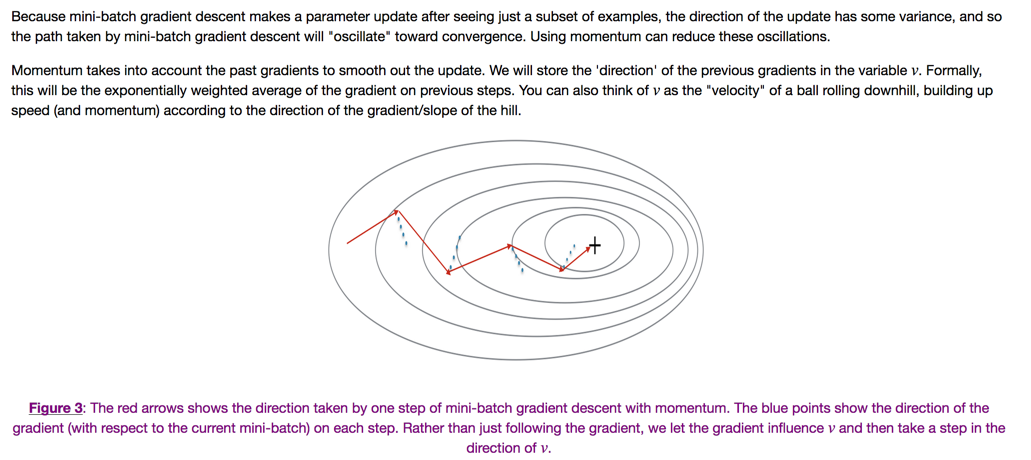$\begin{cases} v_{dW^{[l]}} = \beta v_{dW^{[l]}} + (1 - \beta) dW^{[l]} \\ W^{[l]} = W^{[l]} - \alpha v_{dW^{[l]}} \end{cases}$

$\begin{cases} v_{db^{[l]}} = \beta v_{db^{[l]}} + (1 - \beta) db^{[l]} \\ b^{[l]} = b^{[l]} - \alpha v_{db^{[l]}} \end{cases}$

### Adam optimization algorithm

$\begin{cases} v_{dW^{[l]}} = \beta_1 v_{dW^{[l]}} + (1 - \beta_1) \frac{\partial \mathcal{J} }{ \partial W^{[l]} } \\ v^{corrected}_{dW^{[l]}} = \frac{v_{dW^{[l]}}}{1 - (\beta_1)^t} \\ s_{dW^{[l]}} = \beta_2 s_{dW^{[l]}} + (1 - \beta_2) (\frac{\partial \mathcal{J} }{\partial W^{[l]} })^2 \\ s^{corrected}_{dW^{[l]}} = \frac{s_{dW^{[l]}}}{1 - (\beta_2)^t} \\ W^{[l]} = W^{[l]} - \alpha \frac{v^{corrected}_{dW^{[l]}}}{\sqrt{s^{corrected}_{dW^{[l]}}} + \varepsilon} \end{cases}$

### Learning rate decay

$\lambda$是最主要调的hyperparameter之一。在开始阶段，梯度下降较快，但到了迭代后期，数据已经接近最优解，如果$\lambda$还是过大，可能影响收敛。基于这个思路，迭代到后期时，缩小$\lambda$

$\lambda = {1 \over {1+{decay\_rate}*{epoch\_num}}}$

### Hyperparameter tuning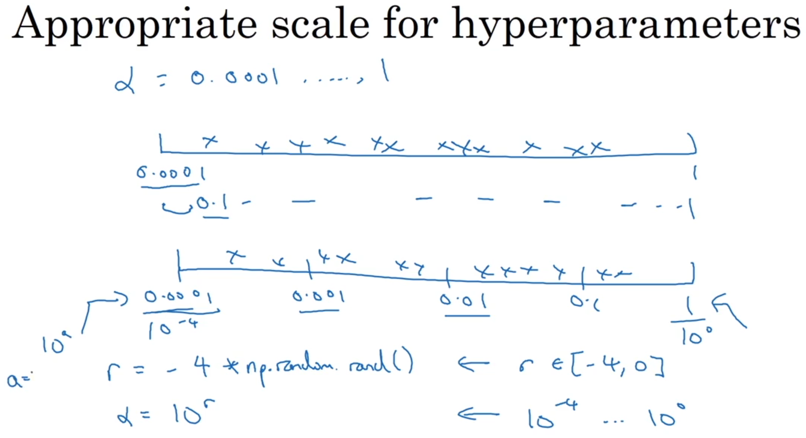## Batch Normalization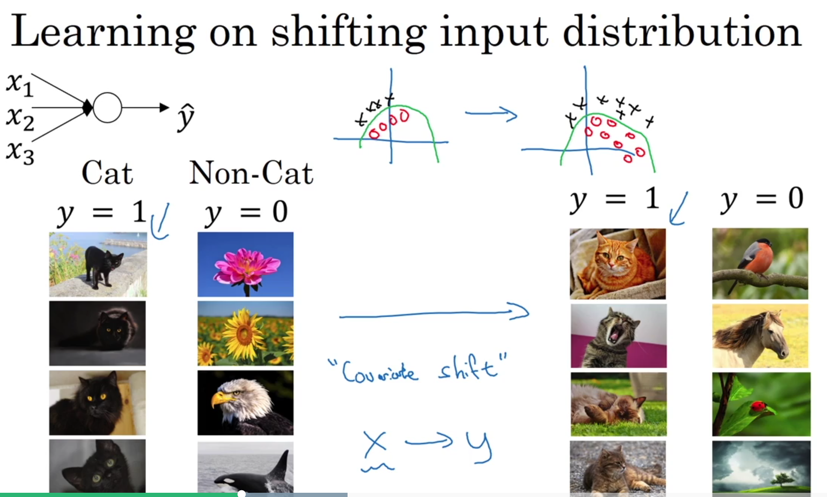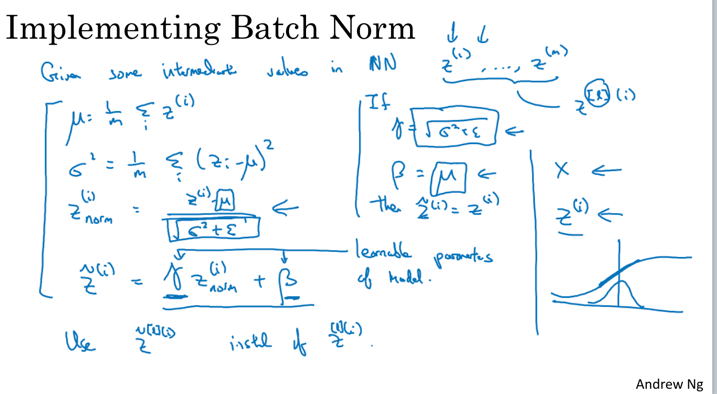• 对m个数据（mini-batchsize）计算得到的$z$统计均值和方差。
• 对数据进行标准化处理。
• 再将数据的均值扩展到$\beta$，方差扩展到$\gamma$。只所以还需要扩展是因为数据的「内部逻辑」本身是有一个分布存在；另外，从激励函数角度考虑，如果数据都是$\mathcal{N}(0, 1)$分布，激励的范围太有限，甚至退化为线性模型。
• 将参数$\gamma$$\beta$放到计算流程中，看做和$W$一样的参数进行求解（注意这里的$\gamma$和momentum中的参数不一样）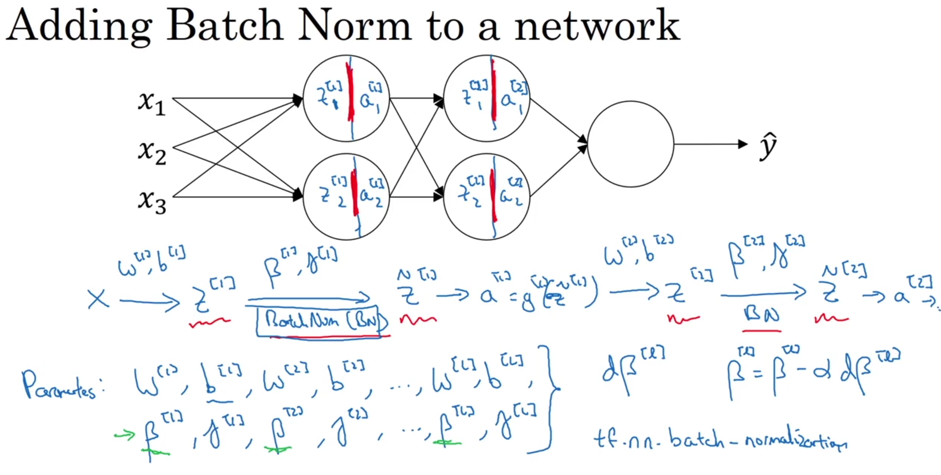## Softmax Regression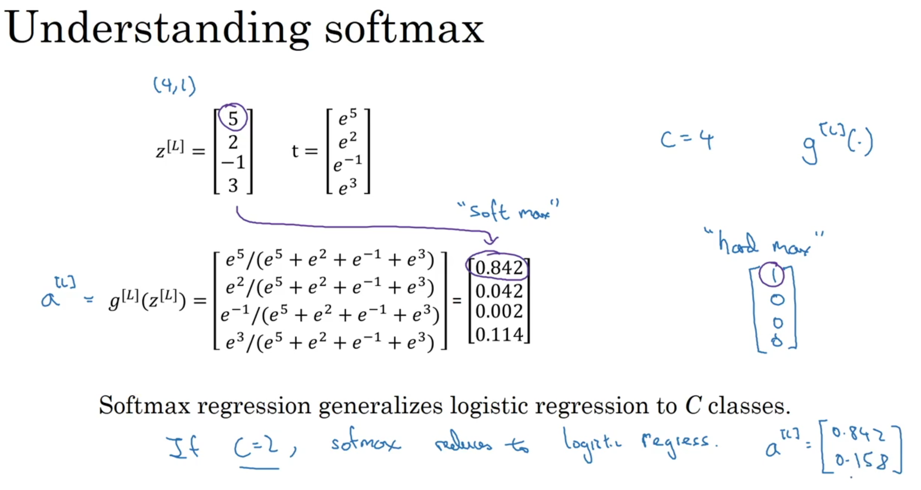softmax的输出结果就是$\hat{y}$，用来统计最后的loss：